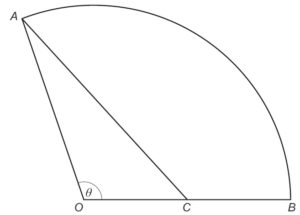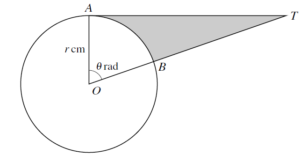# Practice – Non Right-Angled Triangle Trigonometry

1. The sides of a triangle are in arithmetic sequence and the greatest angle is double the smallest angle. Prove that the ratio of its sides is 4:5:6.

2. If  $a\mathrm{cos}A=b\mathrm{cos}B$ , prove that  is either isosceles, or right angled.

3. The angles of a triangle are in the ratio 1:2:7, then show that the ratio of the greatest side to the least side is  .

4. If the angles of a triangle are  $30°$ and  $45°$ and the included side is  $\left(\sqrt{3}+1\right)$ cm, the area of the triangle is  $\frac{1}{2}\left(\sqrt{3}+1\right)$ cm2.

5. The sides of a triangle are  then find the value of the greatest angle of the triangle.

6. The diagram shows a sector of a circle OAB. C is the midpoint of OB. Angle AOB is  $\theta$ radians.Given that the area of the triangle OAC is equal to one quarter of the area of the sector OAB, show that  $\theta =2\mathrm{sin}\theta$ .     [4 marks]

7.The diagram shows a circle with centre O and radius $r$ cm. The points A and B lie on the circle and AT is a tangent to the circle. Angle AOB = 1 radians and OBT is a straight line.
(i) Express the area of the shaded region in terms of  $r$ and $l$ . [3 marks]

(ii) In the case where $r=3$ and $\theta =1.2$ , find the perimeter of the shaded region. [4 marks]